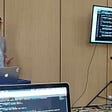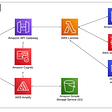# “Big O”?…

I would have to say I was not introduced to ‘Big O Notation’ until after I finished my bootcamp. Big O Notation is very important to understand before getting into algorithms. You will need to know both if you want to perform well on basic coding exams given to you as part of the interview process. It did not take me long to realize something was missing.

Every time I took a test for a company I ran into ?’s…

I was looking at familiar code but the questions being asked to solve the code was beyond me. I’m not sure if anyone else feels this way but I felt completely lost. I tried and failed every time.

There were arrays, strings, functions. However, the way it is worded just completely threw me for a “Loop”. Haha a little coding humor there!

After speaking with multiple engineers, I realized Big O Notation and Algorithms are a huge part of solving the puzzle.

My first go to is Udemy. Why? Well, the more you learn the better. Another way to learn is by watching videos on Youtube.

Either way if you’re new to this coding stuff then you’ll surely want to learn more about Big O.

# What exactly is Big O?

“Measures efficiency of an algorithm”. Why? Large inputs for functions both time & space can cause problems! This is a way to explain to an interviewer how to problem solve. You may get the answer wrong but you may get some points being able to explain how you were trying to solve the problem. First learn about Time and Space.

`Faster = Efficient Performanceknown as O(n)def length(array)  counter = 0  for_in array:  counter += 1. #O(n)  return counterend#The 2nd line of code is Linear Time b/c it has a relationship with the input.  Incrementing by 1.This is 1:1 O(n)`

Every line of code has a Time Complexity.

In Big O, O(n) is Linear Time.

An example (not a great example) of Linear Time :

`[1, 2, 3, 4]#you can pass in an input of size 41 sec.(absolute) 3(input size)This takes 1 Full Second to complete.#Relationship to time to input is 1:1`

Every Variable we create has a space complexity.

`space = memoryextra variables & data structuresdef length(array)  counter = 0  #O(1)  for_in array:  counter += 1  return counterend#First line of code is constant time which has no direct relation with input. O(1)`

Constant Time O(1)

If space & time have no relation with input then it is considered O(1) Constant Time.

# Tiers in Big O

There is an order you can work with in Big O, its from Most to Least efficient.

• O(1) Constant
• O(log n) Logarithmic
• O(n) Linear
• O(n log n) Linear *
• O(2^n) Exponential

It is a good idea to look at each line of code and determine the order. Only the highest is important.

Also, dropping constants to be more clear about what order you are in O(n)? O(1)?

example:

`#if there are 2 loops next to each otherdef addAndCount  counter = 0  total = 0  for_in array:    counter += 1 #O(n)  for_numb in array:    total += number #O(n)  return (counter, total)end#We have two O(n) lines#Both have relationship with input and so it is Linear Time O(n)#Essentially adding n + n = 2n#You can drop the 2 & lean as n b/c of the order `

# Highest Order

In multiple terms only keep the highest order. If you have O(1) + O(n) drop O(1) because of the order.

example:

` if you have O(n) and n = 100  O(100) then   O(n) n = 300 O(n * n) O(300) vs. O(10000) #you're going to go with the highest order.`
• O(1) Constant
• O(log n) Logarithmic
• O(n) Linear
• O(n log n) Linear *
• O(2^n) Exponential

# Keep Learning

This is still a very new concept for me as well. I will definitely take some more time to go over Big O in hopes to better understand Big O notation. Thanks for stopping by and as always Happy Coding!

#CodingEsty

--

--

--

Love podcasts or audiobooks? Learn on the go with our new app.

## What did I learn (as a speaker) from Test Island 2019, Malta’s first testing conference?## Euler Theorem in its core!## 5 Best Android File Manager Apps In 2020## Why You May Want Not Use AWS Amplify for Your Next Serverless Project## TokenStars October Report:  Stability Is A Sign Of Skill## Code Review Essentials## How I was ashamed to be a software tester## let vs var : An underestimated difference in JS world## What is a Pure Function?